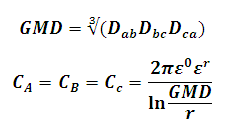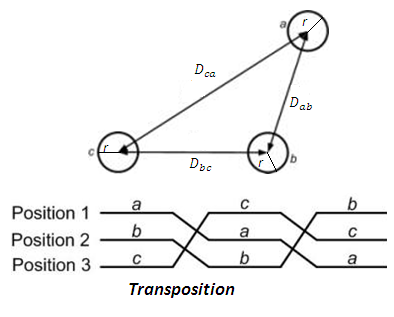# Capacitance of Asymmetric Transposed Three Phase Transmission Line Calculator

This Calctown Calculator calculates the capacitance of an asymmetrically placed transposed three phase transmission line.

Here e0 = 8.854 * 10-12. Please enter er accordingly.

m
m
m
m
unit

#### Result

m
Fwhere

ε0 = Dielectric constant in vaccum

εr = Dielectric ratio

Dab, Dbc, Dca are distances between the lines a, b; b, c; c, a respectively

GMD = Geometric Mean Distance